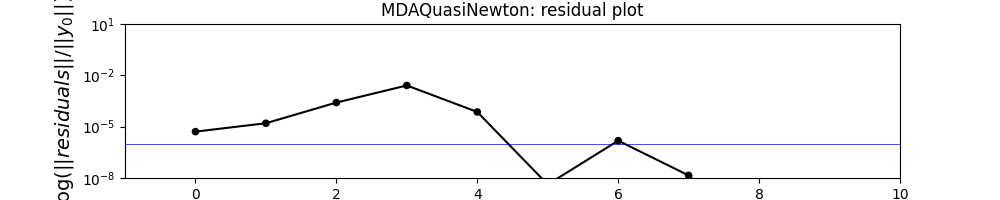# Quasi-Newton MDA¶

from __future__ import division, unicode_literals

from gemseo.api import configure_logger, create_discipline, create_mda

configure_logger()


Out:

<RootLogger root (INFO)>


## Define a way to display results¶

def display_result(res, mda_name):
"""Display coupling and output variables in logger.

@param res: result (dict) of MDA
@param mda_name: name of the current MDA
"""
# names of the coupling variables
coupling_names = [
"y_11",
"y_12",
"y_14",
"y_21",
"y_23",
"y_24",
"y_31",
"y_32",
"y_34",
]
for coupling_var in coupling_names:
print(
"{}, coupling variable {}: {}".format(
mda_name, coupling_var, res[coupling_var]
),
)

# names of the output variables
output_names = ["y_1", "y_2", "y_3", "y_4", "g_1", "g_2", "g_3"]
for output_var in output_names:
print(
"{}, output variable {}: {}".format(mda_name, output_var, res[output_var]),
)


## Create, execute and post-process MDA¶

We do not need to specify the inputs, the default inputs of the MDA will be used and computed from the Default inputs of the disciplines

disciplines = create_discipline(
[
"SobieskiStructure",
"SobieskiPropulsion",
"SobieskiAerodynamics",
"SobieskiMission",
]
)
mda = create_mda("MDAQuasiNewton", disciplines, method="broyden1")
res = mda.execute()
display_result(res, mda.name)
mda.plot_residual_history(
n_iterations=10, logscale=[1e-8, 10.0], show=True, save=False, figsize=(10, 2)
)Out:

 WARNING - 21:49:54: MDAQuasiNewton has reached its maximum number of iterations but the normed residual 1.0 is still above the tolerance 1e-06.
MDAQuasiNewton, coupling variable y_11: [0.15591864]
MDAQuasiNewton, coupling variable y_12: [5.06070636e+04 9.50000000e-01]
MDAQuasiNewton, coupling variable y_14: [50607.06361902  7306.20262124]
MDAQuasiNewton, coupling variable y_21: [50607.06361902]
MDAQuasiNewton, coupling variable y_23: [12194.40917863]
MDAQuasiNewton, coupling variable y_24: [4.15002177]
MDAQuasiNewton, coupling variable y_31: [6354.40199355]
MDAQuasiNewton, coupling variable y_32: [0.50280179]
MDAQuasiNewton, coupling variable y_34: [1.10754577]
MDAQuasiNewton, output variable y_1: [5.06070636e+04 7.30620262e+03 9.50000000e-01]
MDAQuasiNewton, output variable y_2: [5.06070636e+04 1.21944092e+04 4.15002177e+00]
MDAQuasiNewton, output variable y_3: [1.10754577e+00 6.35440199e+03 5.02802105e-01]
MDAQuasiNewton, output variable y_4: [535.78213192]
MDAQuasiNewton, output variable g_1: [ 0.035      -0.00666667 -0.0275     -0.04       -0.04833333 -0.09
-0.15      ]
MDAQuasiNewton, output variable g_2: [-0.04]
MDAQuasiNewton, output variable g_3: [-0.99719789 -0.00280211  0.16206032 -0.02      ]


Total running time of the script: ( 0 minutes 0.262 seconds)

Gallery generated by Sphinx-Gallery